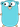# Go 编程：那些隐晦的操作符

``````+    &     +=    &=     &&    ==    !=    (    )
-    |     -=    |=     ||    <     <=    [    ]
*    ^     *=    ^=     <-    >     >=    {    }
/    <<    /=    <<=    ++    =     :=    ,    ;
%    >>    %=    >>=    --    !     ...   .    :
&^          &^=``````## 1. 符号隐晦

``````&    bitwise AND            integers
|    bitwise OR             integers
^    bitwise XOR            integers
&^   bit clear (AND NOT)    integers

<<   left shift             integer << unsigned integer
>>   right shift            integer >> unsigned integer``````

``````package main

import "fmt"

func main(){
fmt.Printf("AND: a(%b) & b(%b) = (%b)\n", 4, 5, (4 & 5))
fmt.Printf("OR:  a(%b) | b(%b) = (%b)\n", 4, 5, (4 | 5))
fmt.Printf("XOR: a(%b) ^ b(%b) = (%b)\n", 4, 5, (4 ^ 5))
fmt.Printf("AND NOT: a(%b) &^ b(%b) = (%b)\n", 4, 5, (4 &^ 5))

fmt.Printf("Left Shift:  a(%b) << 1 = (%b)\n", 5, (5 << 1))
fmt.Printf("Right Shift: a(%b) >> 1 = (%b)\n", 5, (5 >> 1))
}``````

``````AND: a(100) & b(101) = (100)
OR:  a(100) | b(101) = (101)
XOR: a(100) ^ b(101) = (1)
AND NOT: a(100) &^ b(101) = (0)
Left Shift:  a(101) << 1 = (1010)
Right Shift: a(101) >> 1 = (10)``````

``<<=    >>=    &=    ^=   |=``

## 2. 场景隐晦

### 2.1 符号 '_'

• 作为匿名变量赋值使用

``````ar := int{1,2,3,4,5,6,7,8,9,0}
for _, v := range ar {
println(v)
}``````
• 在包引用时使用

``````package YourPackage

import   "lib/math"         //math.Sin
import m "lib/math"         //m.Sin
import . "lib/math"         //Sin``````

``import _ "the/third/pkg"``

``````package main

import _ "github.com/golang/protobuf/protoc-gen-go/grpc"``````

### 2.2 符号 '.'

``````//直接选择属性名或函数名
x.FieldName
x.FunctionName``````

``import . "lib/math"         //Sin``

``````//类型断言: 类型必须用'()'括起来
v, ok := x.(T) ``````

``````//类型转换: 变量必须用'()'括起来
v := T(x)``````

• 类型转换中，待转换的变量`x`只要是一个可以转换成目标类型的变量即可。失败时代码无法编译通过。
• 类型断言中，待断言的变量`x`必须与目标类型一致。如果失败，返回`bool`参数标识。

### 2.3 符号 '...'

``````import "fmt"

func Foo(args ...interface{}) {
for _, arg := range args {
fmt.Println(arg)
}
}``````

``````args := []interface{}{1, false, "hello"}
Foo(args...)``````

``[...]int{1,2,4}``

## 3 特殊符号 '?', 非 Go 语言操作符

``````  import "database/sql"

id := 47
result, err := db.ExecContext(ctx, "UPDATE balances SET balance = balance + 10 WHERE user_id = ?", id)
if err != nil {
log.Fatal(err)
}``````